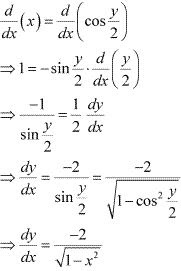# Class 12 Maths NCERT Solutions for Chapter 5 Continuity and Differentiability Exercise 5.3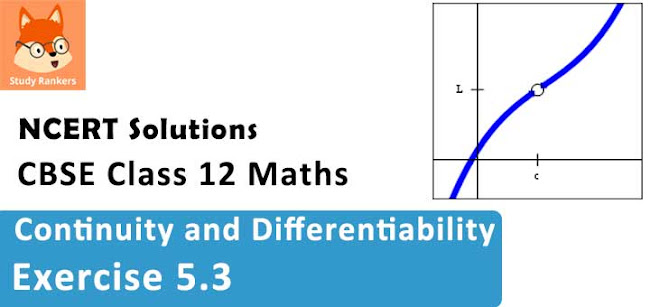### Continuity and Differentiability Exercise 5.3 Solutions

1. Find dy/dx .
2x + 3y = sin x

Solution

The given relationship is 2x + 3y = sin x
Differentiating this relationship with respect to x, we obtain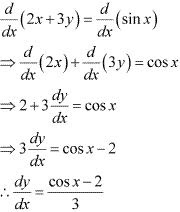2. Find dy/dx
2x + 3y = sin y

Solution

The given relationship is 2x + 3y = sin y
Differentiating this relationship with respect to x, we obtain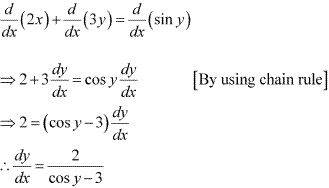3. Find dy/dx
ax + by2 = cos y

Solution

The given relationship is ax + by2 = cos y
Differentiating this relationship with respect to x, we obtain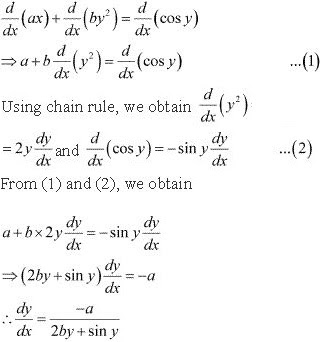4. Find dy/dx
xy + y2 = tan x + y

Solution

The given relationship is xy +  y2 = tan x + y
Differentiating this relationship with respect to x, we obtain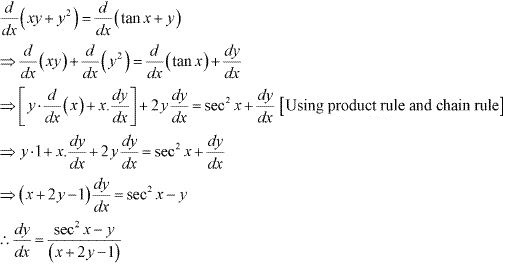5. Find dy/dx
x2 + xy + y2 = 100

Solution

The given relationship is x2 + xy + y2 = 100
Differentiating this relationship with respect to x, we obtain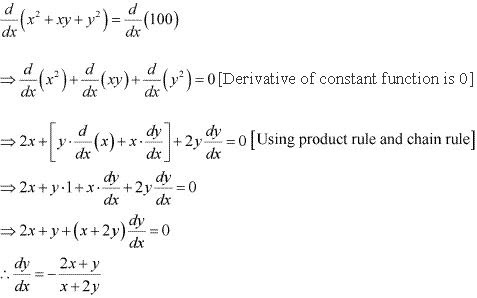6. Find = dy/x
x3 + x2 y + xy2 + y3 = 81
Solution
The given relationship is x3 + x2 y + xy2 + y3 = 81
Differentiating this relationship with respect to x, we obtain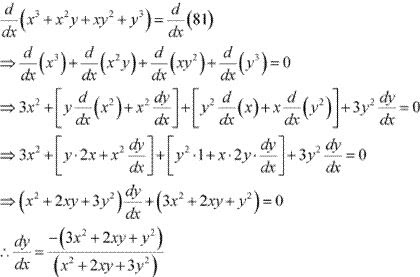7. Find dy/dx
sin2 y + cos xy = π
Solution
The given relationship is sin2 y + cos xy = π
Differentiating this relationship with respect to x, we obtain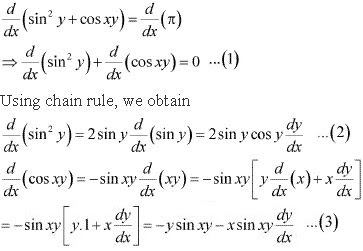From (1), (2) and (3), we obtain
2 sin y cos y dy/dx - y sin xy - (x sin xy) dy/dx = 0
⇒ (2 sin y cos y - x sin xy) dy/dx = y sin xy
⇒ (sin 2y - x sin xy) dy/dx = y sin xy
∴ dy/dx = y sin xy/(sin 2y - x sin xy)

8. Find dy/dx
sin2 x + cos2 y = 1
Solution
The given relationship is sin2 x + cos2 y = 1
Differentiating this relationship with respect to x, we obtain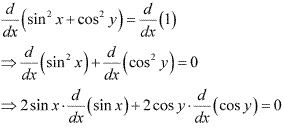⇒ 2 sin x cos x + 2cos y(-sin y). dy/dx = 0
⇒ sin 2x - sin 2y (dy/dx) = 0
∴ dy/dx = sin 2x/sin 2y

9. Find dy/dx
y = sin-1 (2x/(1 + x2 )
Solution
The given relationship is y = sin-1 (2x/(1 + x2 )
y = sin-1 (2x/(1 + x2 )
⇒ sin y = 2x/(1 + x2 )
Differentiating this relationship with respect to x, we obtain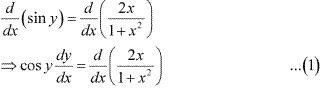The function, 2x/ (1 + x2 ) ,  is of the form of u/v.
Therefore, by quotient rule, we obtain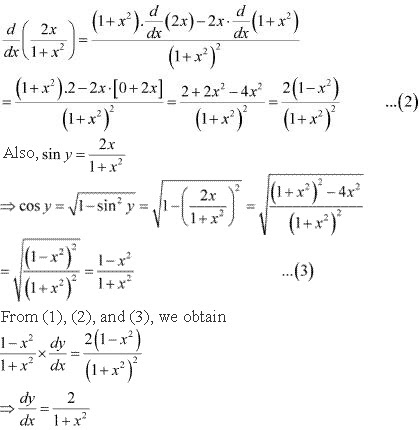10. Find dy/dx
y = tan-1 (3x - x3 )/(1 - 3x2 ), -1/√3 < x < 1/√3
Solution
The given relationship is y = tan-1 (3x - x3 )/(1 - 3x2 ),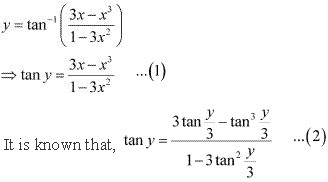Comparing equations (1) and (2), we obtain
x = tan y/3
Differentiating this relationship with respect to x, we obtain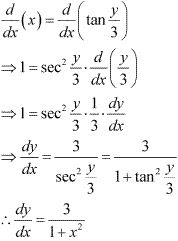11. Find dy/dx
y = cos-1 (1 - x2 )/(1 + x2 ), 0 < x < 1
Solution
The given relationship is,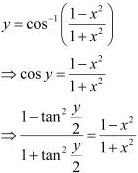On comparing L.H.S. and R.H.S of the above relationship, we obtain
tan y/2 = x
Differentiating this relationship with respect to x, we obtain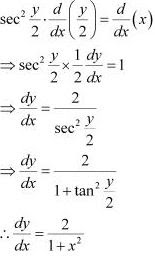12. Find dy/dx
y = sin-1 (1 - x2 )/(1 + x2 ), 0 < x < 1
Solution
The given relationship is y = sin-1 (1 - x2 )/(1 + x2 )
y = sin-1 (1 - x2 )/(1 + x2 )
⇒ sin y = (1 - x2 )/(1 + x2 )
Differentiating this relationship with respect to x, we obtain
d/dx (sin y) = d/dx [(1 - x2 )/(1 + x2 ) ]  ...(1)
Using chain rule, we obtain
d/dx (sin y) = cos y . dy/dx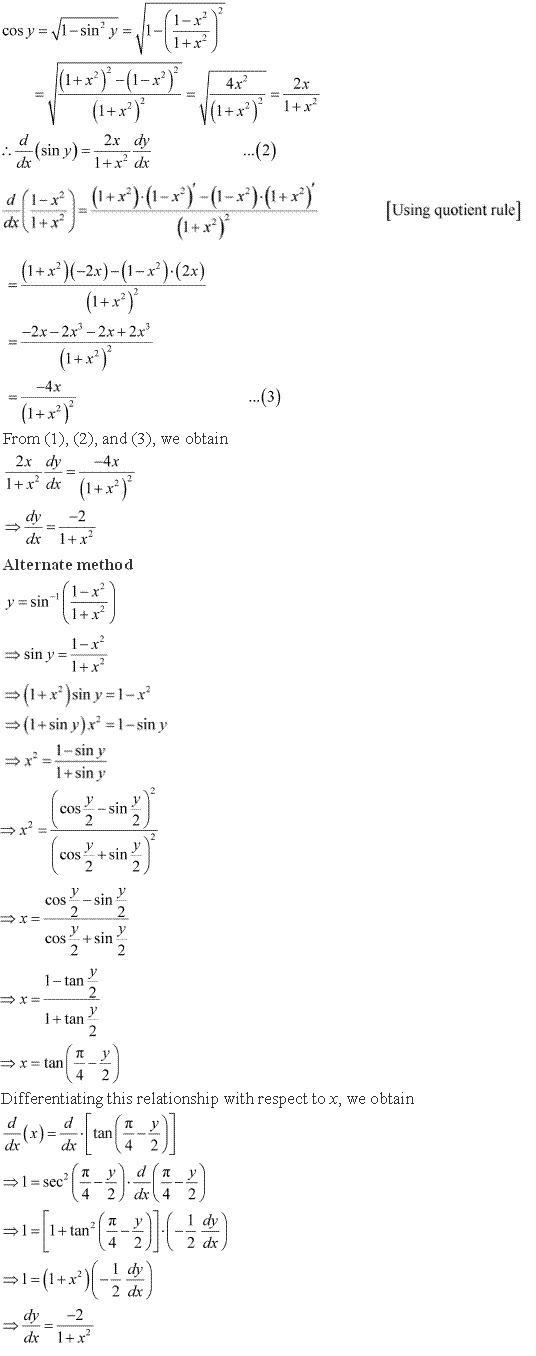13. Find dx/dy
y = cos-1 (2x)/(1 + x2 ), 0 < x < 1
Solution
The given relationship is y = cos-1 (2x)/(1 + x2 )
y = cos-1 (2x)/(1 + x2 )
⇒ cos y = 2x/(1 + x2 )
Differentiating this relationship with respect to x, we obtain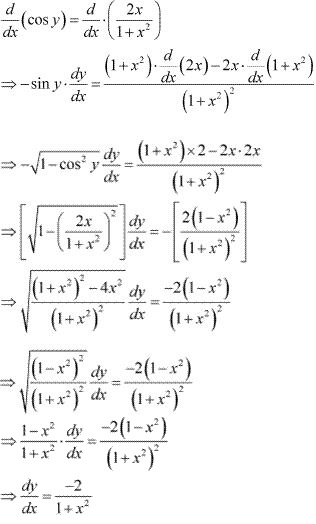14. Find dy/dx
y = sin-1 [2x√(1 - x2 )], -1/√2 < x < 1/√2
Solution
The given relationship is y = sin-1 [2x√(1 - x2 )]
y = sin-1 [2x√(1 - x2 )]
⇒ sin y = 2x√(1 - x2 )
Differentiating this relationship with respect to x, we obtain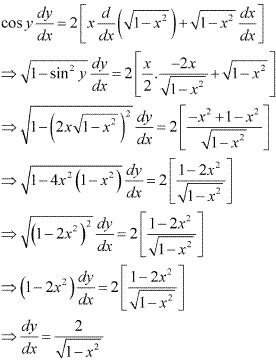15. Find dy/dx
y =  sec-1 [ 1/(2x2 - 1), 0 < x < 1√2
Solution
The given relationship is y = sec-1 [ 1/(2x2 - 1)
y = sec-1 [ 1/(2x2 - 1)
⇒ sec y = 1/(2x2 - 1)
⇒ cos y = 2x2 - 1
⇒ 2x2 = 1 + cos y
⇒ 2x2 = 2 cos2 (y/2)
⇒ x = cos y/2
Differentiating this relationship with respect to x, we obtain